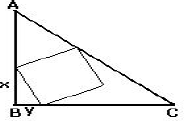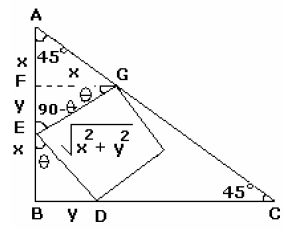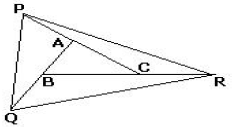# Quantitative Aptitude Quiz for SSC(Mix): 20 March 2021

Updated Sat, 20 Mar 2021 07:31 PM ISTSource: safalta.com
1. A number when divided by a divisor leaves a remainder of 24. When twice the original number is divided by the same divisor, the remainder is 11. What is the value of the divisor ?

### Free Demo Classes

a) 13
b) 37
c) 43
d) 54

Let the original number be 'a'.
Let the divisor be 'd'.
Let the quotient of dividing 'a' by 'd' be 'x'.
Therefore, we can write the division as a/d = x and the remainder is 24.
i.e., a = dx + 24

Twice the original number is divided by d means 2a is divided by d.
We know that a = dx + 24.
Therefore, 2a = 2(dx + 48) or 2a = 2dx + 48
When (2dx + 48) is divided by 'd' the remainder is 11.
2dx is divisible by 'd' and will therefore, not leave a remainder.
The remainder of 11 would be the remainder of dividing 48 by d.
When 37 divides 48, the remainder is 11.
Hence, the divisor is 37

2. If the product of three consecutive positive integers is 15600 then the sum of the squares of these integers is
A) 1777
B) 1785
C) 1875
D) 1877
a (a+1) (a+2) = 15600
The consecutive numbers will be 24, 25 and 26
We have to find,
a^2 + (a+1)^2 + (a+2)^2 = (24)^2 + (25)^2 + (26)^2 = 1877

3. Three consecutive positive even numbers are such that thrice the first number exceeds double the third by 2, then the third number is
a) 10                                   b)14                                       c)16                                        d) 12
Let’s assume no. are 2n, 2n + 2, 2n + 4
3 × 2n = 2(2n + 4) + 2
6n = 4n + 10
n = 5
Third No. = 2n + 4 = 14

4. Atul started a bakery in 1996. Just to note his speed, he did an experiment and noted that he can bake maximum of 26 pizza bases in 78 min. per day. Much to his surprise, he got an order from Domino’s Pizza to supply fresh 18 pizza bases per day. What is the time saved by Atul (in this product only) if the company asked him to supply the pizza bases for the second month of the year only? Assume that he regularly works for the whole month at a constant rate.

a) 10.2 hours
b) 10.6 hours
c) 11.2 hours
d) 11.6 hours

78 min. = 1.3 hours
Speed of Atul = 26/1.3 = 20 pizza bases per hour.
Time taken to bake 18 pizza bases = 18/20 = 9/10 = 0.9 hours
Time saved per day = 1.3 – 0.9 = 0.4 hours
Total time saved during the second month = 29 × 0.4 = 11.6 hours
Note: 1996 is a leap year, no. of days in second month = 29 and not 28

5. A certain positive integer 'N' has exactly 12 distinct divisors, including itself and 1, but has only 3 distinct prime factors. If the sum of these prime factors is 20, then compute the smallest possible value of 'N'.

a) 360
b) 260
c) 308
d) 154

A certain positive integer N has only 3 distinct prime factors
The sum of three prime factors is 20
2 + 5 + 13 = 20 or 2 + 7 + 11 + 20
For the product to be smallest
We take prime 2,5,13
1 x 2 x 5 x 13 = 130 has eight factors
Multiply by 2
We get 260 which has 12 factors

6. In an eating competition sponsored by XYZ ltd, four participants ate chocolates worth Rs. 5.27, Rs. 13.94, Rs. 3.57 and Rs. 1.87, respectively. A fifth participant, who lost the competition, could have eaten chocolates worth how much? [Given that a chocolate costs at least 2 paise.]

a) Rs. 3.91
b) Rs. 2.73
c) Rs. 14.11
d) Rs. 7.66

Let x be the price of 1 chocolate. Clearly x ≥ 2. Now, four participants spend 527,1394, 357, 187(change rs in paise).
So, x should divide 527,1394, 357 & 187. Therefore, x = 17.
Now, number of chocolates eaten by participants: 527/17 = 31, 1394/17 = 82, 357/17 = 21, 187/17 = 11.
Now, fifth participant has lost the competition. So, he must have eaten less than 82 chocolates. So, answer should be multiple of 17 and must give ≤ 82, when divided by 17. Now, 1st and 3rd options are multiples of 17. But, 1411/17 = 83. So, answer will be 1st option as 391/17 = 23 which is less than 82.

7. How many pairs of positive integers, not more than 100, will have an average greater than 50?

a) 1275
b) 2550
c) 2250
d) 2500

Average of 2 numbers is greater than 50 means that the sum should be greater than 100.
Different pairs are as follows:
(1, 100) … (1 pair)
(2, 99), (2, 100) … (2 pairs)
(3, 98), (3, 99), (3, 100) … (3 pairs)
... so on
(49, 52), (49, 53) ... (49, 100) ... (49 pairs)
(50, 51), (50, 52) … (50, 100) ... (50 pairs)
So, 1 + 2 + 3 + 4 + ... + 50 = 1275
Again
(51, 51), (51, 52), (51, 53), ... (51, 100) ... (50 pairs)
(52, 52), (52, 53), (52, 54), ... (52, 100) ... (49 pairs)
(53, 53), (53, 54), (53, 55), ... (53, 100) ... (48 pairs)
... so on
(99, 99), (99, 100) ... (2 pairs)
(100, 100) ... (1 pair)
So, 1 + 2 + 3 + 4 + ... + 50 = 1275
Hence, total = 1275 + 1275 = 2550 pairs

8. A family consists of a grandfather, 6 sons and daughters and 5 grand children. They are to be seated in a row for dinner. The grand children wish to occupy the two seats at each end and the grandfather refuses to have a grandchild on either side of him. In how many ways can the seating arrangements be made for the dinner?
There are 6 adults, 4 grandchildren and 1 grandfather.

Let us mark the seat for 11 persons from 1 to 11.
S S S S S S
1 2 3 .....................9 10 11
Seats number 1, 2 and 10, 11 at the ends are to be occupied by 4 grand children and it can
be done in 4P4 = 4! = 24 ways.
Now, we will seat the grandfather who cannot occupy seat number 3 or seat number 9
because he does not want to have a child by his side. Hence, he has to choose any of five
seats 4, 5, 6, 7, 8 i.e. can seat himself in 5 ways.

9. ABC is an isosceles triangle right angled at B. A square is inscribed inside the triangle with three vertices of the square on three sides of the triangle as shown in the adjacent figure. It is known that the ratio x to y is equal to 2 to 1. The ratio of the area of the square to the area of the triangle is equal to(a) 2: 5                  (b) 1: 10                               (c) 1: 3                                 (d) 2: 3

We draw a perpendicular GF from the vertex G of the square on the
side AB. ΔEFG and ΔEBD are congruent (three angles equal and one side
equal)
⇒ EF = y and FG = x
Now FG = AF (∠FAG = ∠AGF = 450)10. In triangle ABC, sides AB, AC, and BC are extended till Q, P and R such that AC = AP, BC = CR, and AB = BQ, as shown in the adjacent figure. It is known that the area of triangle ABC is 10 square centimetres.What is the area of triangle PQR?

(a) 40 cm2                            (b) 70 cm2                            (c) 80 cm2                            (d) 90 cm2

Let’s join A to R, B to P, and C to Q. The median divide the triangle into two equal areas. QC, AR, BP are medians in also ΔQBR, ΔPCR and ΔAPQ, Also PC, BR, AQ are medians in triangle PBR, AQR, PQC. Equating the areas formed by medians, we can see Area ΔPQR = 7area ΔABC = 70cm^2

### Disclaimer

अपनी वेबसाइट पर हम डाटा संग्रह टूल्स, जैसे की कुकीज के माध्यम से आपकी जानकारी एकत्र करते हैं ताकि आपको बेहतर अनुभव प्रदान कर सकें, वेबसाइट के ट्रैफिक का विश्लेषण कर सकें, कॉन्टेंट व्यक्तिगत तरीके से पेश कर सकें और हमारे पार्टनर्स, जैसे की Google, और सोशल मीडिया साइट्स, जैसे की Facebook, के साथ लक्षित विज्ञापन पेश करने के लिए उपयोग कर सकें। साथ ही, अगर आप साइन-अप करते हैं, तो हम आपका ईमेल पता, फोन नंबर और अन्य विवरण पूरी तरह सुरक्षित तरीके से स्टोर करते हैं। आप कुकीज नीति पृष्ठ से अपनी कुकीज हटा सकते है और रजिस्टर्ड यूजर अपने प्रोफाइल पेज से अपना व्यक्तिगत डाटा हटा या एक्सपोर्ट कर सकते हैं। हमारी Cookies Policy, Privacy Policy और Terms & Conditions के बारे में पढ़ें और अपनी सहमति देने के लिए Agree पर क्लिक करें।

Agree

Get free counselling session